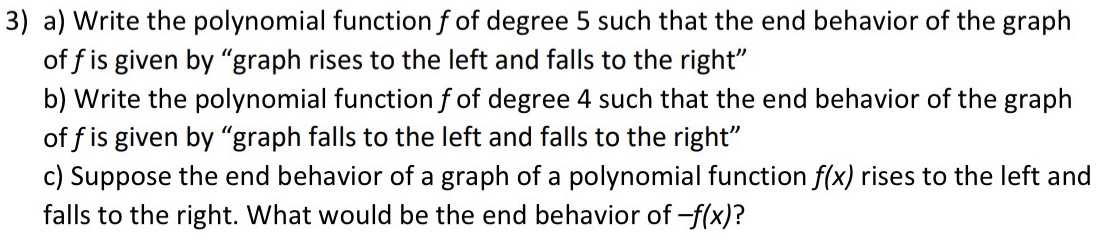### ¿Todavía tienes preguntas de matemáticas?

Pregunte a nuestros tutores expertos
Algebra
Pregunta3) a) Write the polynomial function $$f$$ of degree $$5$$ such that the end behavior of the graph of $$f$$ is given by "graph rises to the left and falls to the right" b) Write the polynomial function $$f$$ of degree $$4$$ such that the end behavior of the graph of $$f$$ is given by "graph falls to the left and falls to the right" c) Suppose the end behavior of a graph of a polynomial function $$f ( x )$$ rises to the left and falls to the right. What would be the end behavior of $$- f ( x )$$ ?

a) f(x)=-2x$$^5$$
b) f(x)=-3x$$^4$$